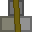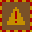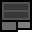# Account 789542

## BalanceMetal: 0s0 = 0Explosives: 72s0 = 1152RC Turrets: 0

## Transfer

 PIN Receiver ReferenceMetal 16 × +Explosives 16 × +RC Turrets

## Create new account token

PIN:

New accounts cost 2 stacks (32 units) metal. You must give the token directly to the other player. Do not create the account yourself and give them the details. If you are found to do this, your account will be closed and all your items taken. You are responsible for ensuring the player you provide a new account token to knows how to use dredbank properly. If many players are found who don't know how to use dredbank properly, and they all got their accounts from one player, there may be a punishment against the player who provided their accounts.

## Transactions

Type Counterparty Account Reference NumberMetalExplosivesRC Turrets
Sent 220022 0 16s8 = 264 0s0 = 0 0
Sent 852133 0 25s0 = 400 0s0 = 0 0
Deposit 0 0s0 = 0 11s0 = 176 0
Deposit 0 0s0 = 0 10s0 = 160 0
Received 852133 8888 0s0 = 0 5s0 = 80 0
Received 852133 8888 0s0 = 0 19s0 = 304 0
Sent 852133 1000 0s8 = 8 0s0 = 0 0
Received 852133 8888 1s0 = 16 2s0 = 32 0
Received 483744 0 9s0 = 144 5s0 = 80 0
Received 483744 1000 9s0 = 144 4s0 = 64 0
Received 483744 1000 4s0 = 64 2s0 = 32 0
Sent 653090 0 0s15 = 15 0s0 = 0 0
Received 984526 0 6s0 = 96 1s0 = 16 0
Deposit 0 3s0 = 48 2s0 = 32 0
Deposit 0 10s0 = 160 0s0 = 0 0
Deposit 0 0s0 = 0 8s0 = 128 0
Deposit 0 0s0 = 0 3s0 = 48 0
Sent 653090 0 0s1 = 1 0s0 = 0 0
Deposit 0 1s0 = 16 0s0 = 0 0